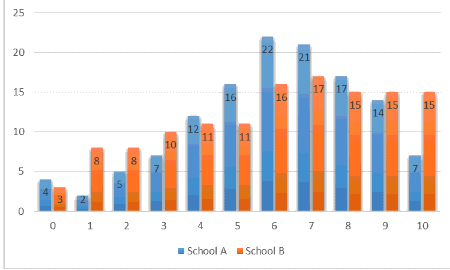# Statistical Questions

A statistical question is one for which you don't expect to get a single answer. Instead, you expect to get a variety of different answers, and you are interested in the distribution and tendency of those answers.

For example, "How tall are you?" is not a statistical question. But "How tall are the students in your school?" is a statistical question.

Central Tendency

When the answers to a statistical question are numerical data, we can ask about the central tendency of that data. For example, we might want to know, roughly, "how tall are most people in your school?"

However, this is not a precise question. There are ways of measuring of central tendency using a single number: you can find the mean (or average), the median, or the mode of the data.

Variation

Suppose you know that the average height of a student in a school is $5$ feet. You may still be interested in the spread of the data. Is everyone nearly $5$ feet tall, say within $2$ inches? Or are there also very short and very tall people in the school?

The simplest measure of the variation is the range , calculated by subtracting the lowest value from the highest. More complex measures of variation include the interquartile range and the standard deviation or variance .

Below is a graph of two data sets, showing the number of students in two schools that got a particular score on a $10$ -problem quiz.Both data sets have the same range ( $10$ ), the same mean (a little more than $6$ ), and the same total number of students (you can't tell this easily from the graph, but it's true.) However, the heights of the blue blocks vary considerably compared to that of red blocks.